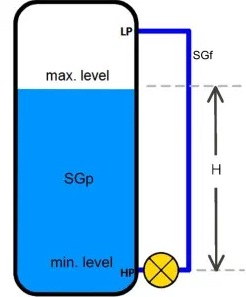# DRY & WET LEG LEVEL MEASUREMENT### Introduction

There are so many level measuring techniques in present.one of the method is measuring level with the help of DP transmitter.There are 2 methods ;Dry & wet leg level measurement this session we are gonna discuss about that

### Dry Leg System

A full dry leg installation with three-valve manifold is shown in Figure below. If the gas phase is condensable, say steam, condensate will form in the low-pressure impulse line resulting in a column of liquid, which exerts extra pressure on the low-pressure side of the transmitter.

A technique to solve this problem is to add a knockout pot below the transmitter in the low-pressure side as shown in Figure. Periodic draining of the condensate in the knockout pot will ensure that the impulse line is free of liquid.

In practice, a dry leg is seldom used because frequent maintenance is required. One example of a dry leg application is the measurement of liquid poison level in the poison injection tank, where the gas phase is no condensable helium. In most closed tank applications, a wet leg level measurement system is used### Wet Leg System

In a wet leg system, the low-pressure impulse line is completely filled with liquid (usually the same liquid as the process) and hence the name wet leg.A level transmitter, with the associated three-valve manifold, is used in an identical manner to the dry leg system At the top of the low pressure impulse line is a small catch tank. The gas phase or vapour will condense in the wet leg and the catch tank. The catch tank, with the inclined interconnecting line, maintains a constant hydrostatic pressure on the low-pressure side of the level transmitter. This pressure, being a constant, can easily be compensated for by calibration.

If the tank is located outdoors, trace heating of the wet leg might be necessary to prevent it from freezing. Steam lines or an electric heating element can be wound around the wet leg to keep the temperature of the condensate above its freezing point.

Note the two sets of drain valves. The transmitter drain valves would be used to drain (bleed) the transmitter only. The two drain valves located immediately above the three-valve manifold are used for impulse and wet leg draining and filling.

###Span = (X) (GL)

HW at minimum level = (Z) (GS) + (Y) (GL)

HW at maximum level  = ( Z ) ( GS )  +  ( X + Y ) ( GL )

Where:

G= Specific gravity of tank liquid.

GS   = Specific gravity of seal liquid.

HW = Equivalent head of water.

X, Y & Z are shown

Open tank with X   = 300 inches

Y    = 50 inches

Z    = 10 inches

GL =   0.8

GS =   0.9

Span   = (300) (0.8) = 240 inches

HW at minimum level =   (10) (0.9) + (50) (0.8) = 49 inches

HW at maximum level = (10) (0.9) + (50 + 300) (0.8) = 289 inches

Calibrated range = 49 to 289 inches head of water

#### Close tank with wet leg:

Span   = (X) (GL)

###HW at minimum level    = (Y) (GL) – (d)(GS)

HW at maximum level   = (X + Y) (GL) – (d) (GS)

Where: GL = Specific gravity of tank liquid

GS = Specific gravity of tank liquid

HW   = Equivalent head of water

X, Y and Z are shown below

X = 300 inches

Y = 50 inches

d = 500 inches

GL = 0.8

GS = 0.9

Span = (300) (0.8) = 240 inches

HW minimum level = (50) (0.8)  –  (500) (0.9)  =  – 410 inches

HW maximum level = (300 + 50) (0.8) – (500) (0.9) = – 170 inches

Calibrated range = – 410 to –170 inches head of water.

(Minus sings indicate that the higher pressure is applied to the low pressure side of the transmitter

To know about other level measuring techniques like  LASER LEVEL MEASURMENTUltrasonic  level Measurement

Scroll to Top